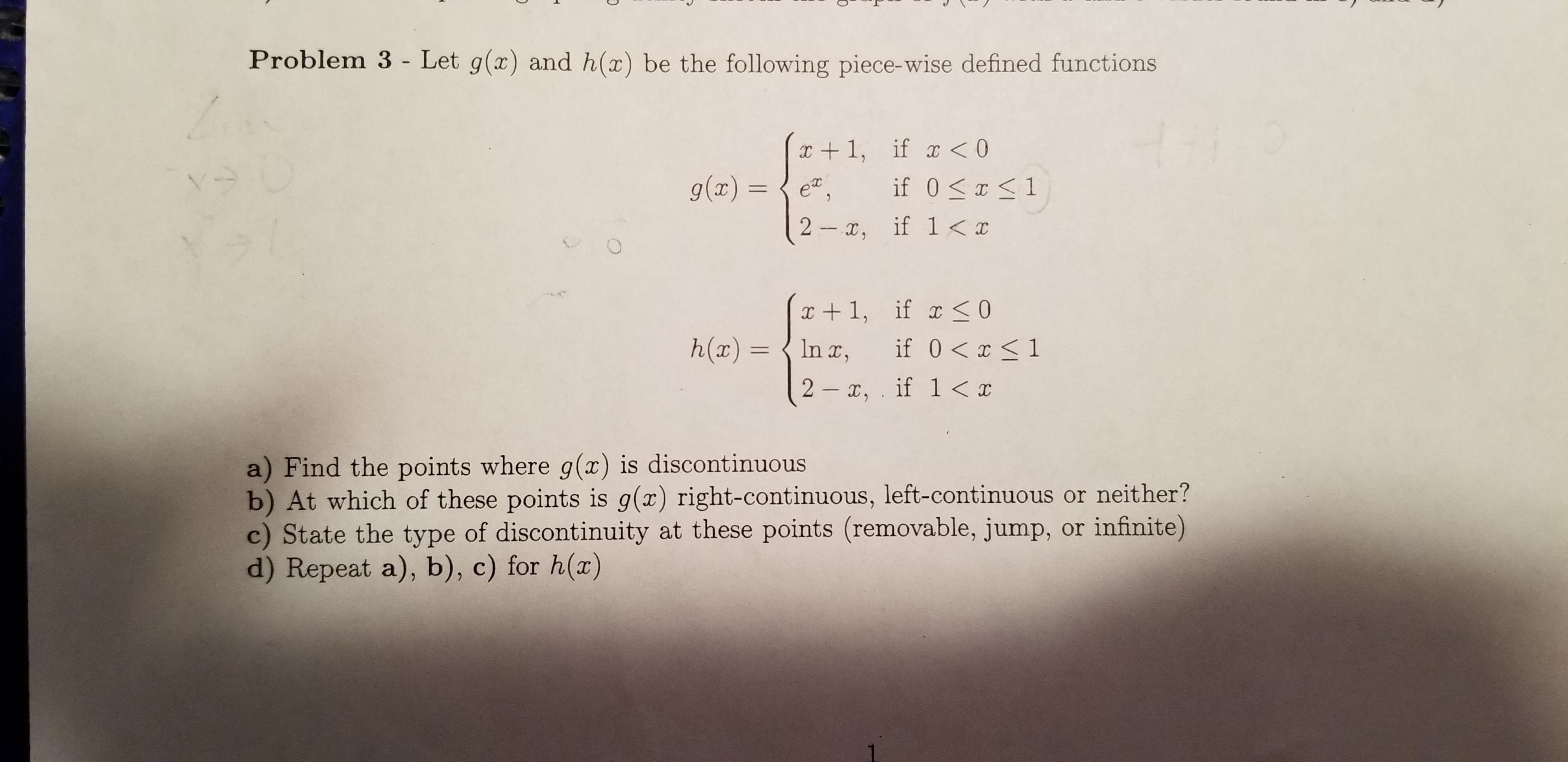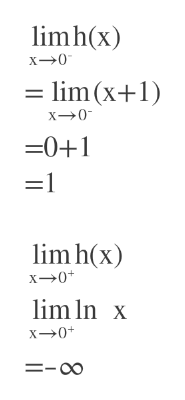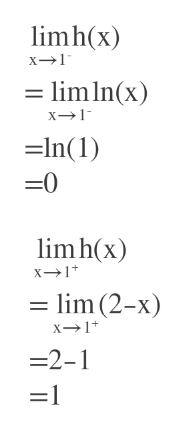Problem 3 - Let g(x) and h(x) be the following piece-wise defined functionsx1, if x

Questionhelp_outlineImage TranscriptioncloseProblem 3 - Let g(x) and h(x) be the following piece-wise defined functions x1, if x<0 if 0 1 g(ax) IC 2 , if 1
Step 1

Left hand limit of x=0 is=0+1=1

Right hand limit of x=0 is= -infinity

So, at x=0 it is discontinuoushelp_outlineImage Transcriptioncloselimh(x) x-0 = lim (x+1) X0 -0+1 lim h(x) x-0 lim In x x0 =-oo fullscreen
Step 2

Left hand limit of x=1 is =0

Right hand limit of x=1 is = 1

So h(x) is discontinuous at x=1

Answer(a): h(x) is discontinuous at x=0 and x=1help_outlineImage Transcriptioncloselimh(x) x1 limln(x) x-1 =In(1) =0 lim h(x) x1 =lm (2-x) x1 -2-1 =1 fullscreen
Step 3

Both at x=0 and x=1 it is left continuous , because equality holds at left...

Want to see the full answer?

See Solution

Want to see this answer and more?

Our solutions are written by experts, many with advanced degrees, and available 24/7

See Solution
Tagged in

Continuity